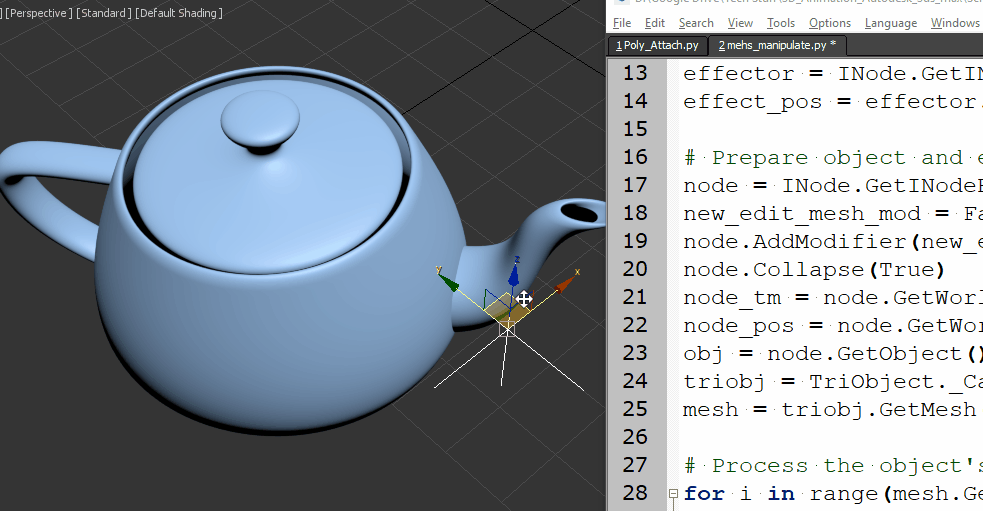## Blender – Python – Access animated vertices data

Software:
Blender 2.83

The following is simple example of reading a mesh’s animated vertices data.

This example code gist

Note that accessing an model’s animated vertex locations requires reading the model’s evaluated (deformed) mesh state per frame.
For that reason a new bmesh object is initiated per each frame with the the model’s updated dependency graph.

``````import bpy
import bmesh
obj = bpy.context.active_object
frames = range(0,10)
get the object's evaluated dependency graph:
depgraph = bpy.context.evaluated_depsgraph_get()
iterate animation frames:
for f in frames:
bpy.context.scene.frame_set(f)
# define new bmesh object:
bm = bmesh.new()
bm.verts.ensure_lookup_table()
# read the evaluated mesh data into the bmesh   object:
bm.from_object( obj, depgraph )
# iterate the bmesh verts:
for i, v in enumerate(bm.verts):
print("frame: {}, vert: {}, location: {}".format(f, i, v.co))``````

## Blender – Vertex Paint Selected Vertices

Software:
Blender 2.82

1. In Edit Mode, Select the wanted vertices.
2. In Vertex Paint Mode,
Activate the Vertex Selection option:3. Use the brush to paint or Shift + K to set the selected vertices color.

## Blender Python – Accessing mesh triangles

Software:
Blender 2.81 | Python 3.74

By default, mesh triangles are not accessible to Python in Blender.
When accessing the mesh triangles is needed, they must be calculated first using the ‘calc_loop_triangles’ Mesh object method.
Before the calc_loop_triangle method has been called, the loop_triangles property of the Mesh object will reference a empty collection.
After calling the calc_loop_triangles method, the loop_triangles property will reference a collection of MeshLoopTriangle objects, in which the vertices property will hold an array of 3 integers that are the indices of the triangle vertices.

The following example script creates and places sphere objects at the centers of the cube’s triangles:

```import bpy
mesh = bpy.data.objects['Cube'].data
mesh.calc_loop_triangles()
for tri in mesh.loop_triangles:
tri_center = (mesh.vertices[tri.vertices].co * 0.333) +\
(mesh.vertices[tri.vertices].co * 0.333) +\
(mesh.vertices[tri.vertices].co * 0.333)
enter_editmode=False,
location=tri_center)```

* note that when copying and pasting a script from this example, the indentation may not be pasted correctly.

## Python for 3ds max – Mesh manipulation

Software:
3ds max 2019

An example of creating a mesh ripple deformation using Python for 3ds max:Script steps:

1. Define the effect intensity and a helper point object that will serve as the effect center.
2. Collapse the object to an Editable-Mesh so its vertices will be accessible by Python.
Note that the Node‘s Object has to be cast as a TriObject, to access the object’s Mesh data.
3.  Loop through the Mesh’s vertices, get their world position, and set a new Z position as a sine function of the distance from the effect center.
```import math
from MaxPlus import Factory
from MaxPlus import ClassIds
from MaxPlus import INode
from MaxPlus import TriObject
from MaxPlus import Matrix3
from MaxPlus import Point3

# Intensity:
effecr_mult = 1.0

# Effect center:
effector = INode.GetINodeByName('Point001')
effect_pos = effector.GetWorldPosition()

# Prepare object and eccess it's mesh data:
node = INode.GetINodeByName('Teapot001')
new_edit_mesh_mod = Factory.CreateObjectModifier(ClassIds.Edit_Mesh)
node.Collapse(True)
node_tm = node.GetWorldTM()
node_pos = node.GetWorldPosition()
obj = node.GetObject()
triobj = TriObject._CastFrom(obj)
mesh = triobj.GetMesh()

# Process the object's vertices:
for i in range(mesh.GetNumVertices()):
# Get vertex in world space
vert_pos = mesh.GetVertex(i)
vert_world_pos = node_tm.VectorTransform(vert_pos)
vert_world_pos = vert_world_pos + node_pos
# Get vertex distance from effect center:
diff_vec = vert_world_pos - effect_pos
diff_vec.Z = 0
dist = diff_vec.GetLength()
# Set new vertex position:
mesh.SetVert(i,vert_pos.X,vert_pos.Y,vert_pos.Z + math.sin(dist)*effecr_mult)```

* note that when copying and pasting a script from this example, the indentation may not be pasted correctly.

Related Post:
Python for 3ds max – Animated Mesh

## Python for 3ds max – Animated Mesh

Software:
3ds max 2019

This is an example of procedurally animating a mesh’s vertices via Python script.Notes:
1. The model has to be converted to Editable Mesh before the script is run.
* unless the scrip will be extended to do it.
2. The model must be selected for the script to work.

```import MaxPlus
import math
from MaxPlus import INode
from MaxPlus import TriObject
from MaxPlus import SelectionManager
from MaxPlus import Factory
from MaxPlus import Animation
from MaxPlus import Point3
from MaxPlus import Control

ticks_frame = 160

#Selection
sel = []
for n in SelectionManager.Nodes:
sel.append(n)
node = sel

#Setup Controllers
obj = node.GetObject()
Tri = TriObject._CastFrom(obj)
mesh = Tri.GetMesh()
num_verts = mesh.GetNumVertices()
mesh_anim = obj.GetSubAnim(0)
pnt_ctrl = Factory.CreateDefaultMasterPointController()
node.AssignController(pnt_ctrl,1)
for i in range(num_verts):
bezp3 = Factory.CreateDefaultPoint3Controller()
bezp3.SetPoint3Value(mesh.GetVertex(i))
mesh_anim.AssignController(bezp3,i)

#Animation
Animation.SetAnimateButtonState(True)
for t in range(100):
time = t * ticks_frame
Animation.SetTime(time)